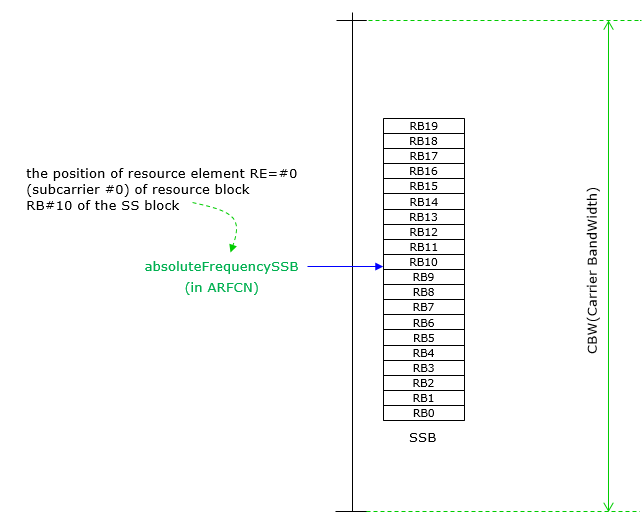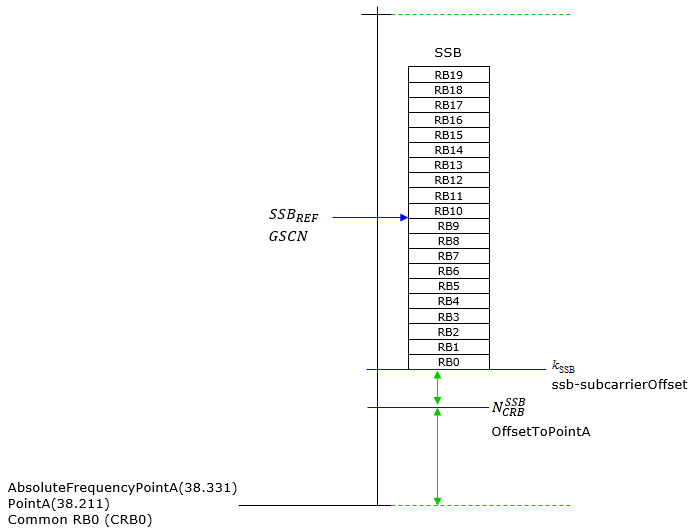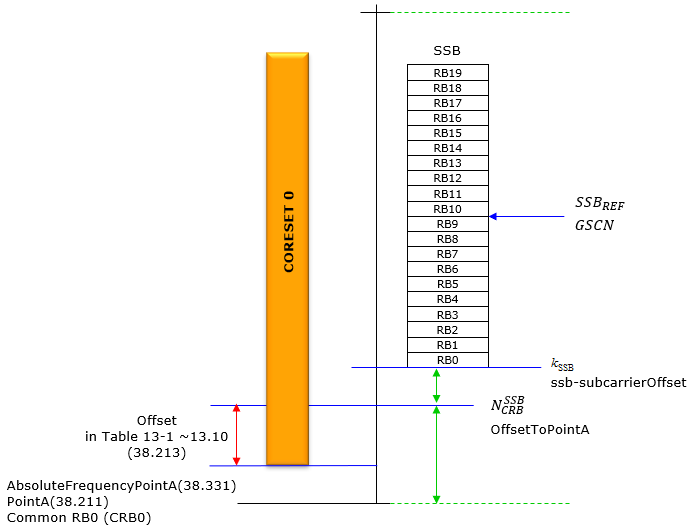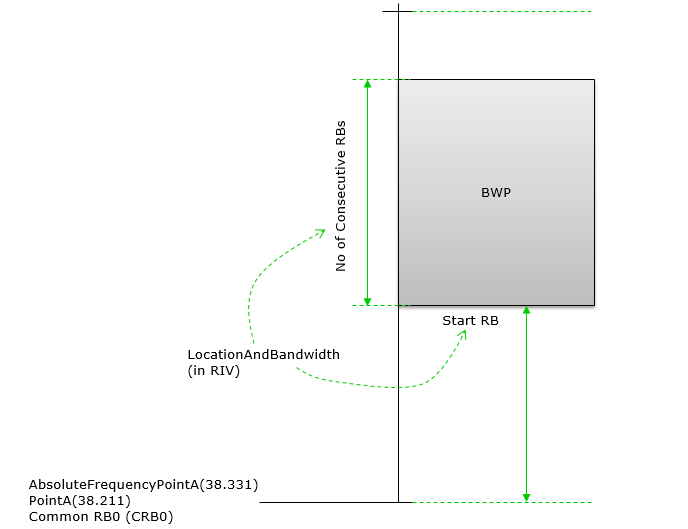5G/NR - Resource Block Indexing                                   Home : www.sharetechnote.com Resource Block Indexing / Frequency Domain Location   As you know, NR use various types of subcarrier spacing (Numerology). In addition, it can use different subcarrier spacing within the same channel bandwidth depending on type of channel/signal (e.g, SSB, PDSCH etc) or BWP. Then the problem (confusing part) is how to specify the location of each resource blocks using differentsubcarrier spacing. We need some kind of reference coordinate system for this.       Reference Subcarrier Spacing for FR1 and FR2   In NR, there are two different type of reference coordinate system and these reference coordinate system is called Reference PRB. In sub 6 Ghz(FR1), we use the reference PRB system based on 15 Khz subcarrier spacing and in mmWave(FR2) we use the reference PRB sysbem based on on 60 khz subcarrier spacing as illustrated below.Point A and Offset   This kind of common reference point shown above is represented as a specific term named as 'PointA' in 3GPP. The PointA is defined in 38.211 - 4.4.4.2 as follows. Point A serves as a common reference point for resource block grids and is obtained from: offsetToPointA for a PCell downlink represents the frequency offset between point A and the lowest subcarrier of the lowest resource block of the SS/PBCH block used by the UE for initial cell selection, expressed in units of resource blocks assuming 15 kHz subcarrier spacing for FR1 and 60 kHz subcarrier spacing for FR2; absoluteFrequencyPointA for all other cases where absoluteFrequencyPointA represents the frequency-location of point A expressed as in ARFCN.   For example, let's assume that we configure SSB with 30 Khz subcarrier spacing in FR1. We can configure the frequency domain location of SSB in Reference PRB coordinate as shown below and the position can be informed to UE via signaling message.SSB Frequency Location in NSA/ENDC   The illustration shown below is based on the following statement on absoluteFrequencySSB from 38.331. As you may notice, there is slight differences between the two versions. The biggest difference is the part in red. The illustration shown below is based on v15.2.1.   38.331 V15.2.1 (2018-06) Frequency of the SSB to be used for this serving cell. The frequency provided in this field identifies the position of resource element RE=#0 (subcarrier #0) of resource block RB#10 of the SS block. The cell-defining SSB of the PCell is always on the sync raster. Frequencies are considered to be on the sync raster if they are also identifiable with a GSCN value (38.101). If the field is absent, the SSB related parameters should be absent, e.g. ssb-PositionsInBurst, ssb-periodicityServingCell and subcarrierSpacing in ServingCellConfigCommon IE. If the field is absent, the UE obtains timing reference from the SpCell. This is only supported in case the Scell is in the same frequency band as the SpCell.   38.331 V15.5.1 (2019-05) Frequency of the SSB to be used for this serving cell. SSB related parameters (e.g. SSB index) provided for a serving cell refer to this SSB frequency unless mentioned otherwise. The cell-defining SSB of the PCell is always on the sync raster. Frequencies are considered to be on the sync raster if they are also identifiable with a GSCN value(TS 38.101-1). If the field is absent, the SSB related parameters should be absent, e.g. ssb-PositionsInBurst, ssb-periodicityServingCell and subcarrierSpacing in ServingCellConfigCommon IE. If the field is absent, the UE obtains timing reference from the SpCell. This is only supported in case the Scell is in the same frequency band as the SpCell.PointA is defined in 38.211 - 4.4.4.2 as follows. Point A serves as a common reference point for resource block grids and is obtained from: offsetToPointA for a PCell downlink represents the frequency offset between point A and the lowest subcarrier of the lowest resource block of the SS/PBCH block used by the UE for initial cell selection, expressed in units of resource blocks assuming 15 kHz subcarrier spacing for FR1 and 60 kHz subcarrier spacing for FR2; absoluteFrequencyPointA for all other cases where absoluteFrequencyPointA represents the frequency-location of point A expressed as in ARFCN.   AbsoluteFrequencyPointA is defined by 38.331 (v15.7) as follow. Absolute frequency position of the reference resource block (Common RB 0). Its lowest subcarrier is also known as Point A . Note that the lower edge of the actual carrier is not defined by this field but rather in the scs-SpecificCarrierList.   GSCN is with reference to the center frequency of SSB based on the statement in 38.104 - 5.4.3.2. The mapping between the synchronization raster and the corresponding resource element of the SS block is given in table 5.4.3.2-1. The mapping depends on the total number of RBs that are allocated in the channel and applies to both UL and DL. < 38.104 v15.7 - Table 5.4.3.2-1: Synchronization Raster to SS block Resource Element Mapping >I assume that both GCSN and SSB_REF are with the reference to the center frequency of SSB based on the statement in 38.104 - 5.4.3.2. The frequency position of the SS block is defined as SS_REF with corresponding number GSCN. The parameters defining the SSREF and GSCN for all the frequency ranges are in table 5.4.3.1-1 < 38.104 v15.7 - Table 5.4.3.1-1: GSCN parameters for the global frequency raster >Putting all the statements mentioned above, I can illustrate the SSB position and PointA, OffsetToPointA,ssb-subcarrierOffset as follows.Example 01 > PointA Calculation for SCS 30Khs   Assuming followings are given (1) GSCN (ARFCN) (2) k_SSB (3) OffsetToPointA Step 1 :  Convert (1) into the frequency in Mhz and assign it to (A) Step 2 :  Calculate the SSB Reference Location(subcarrier 0 in RB0 of SSB) in Mhz and store it to (B) (B) = (A) - (10 x 12 x 0.03)   NOTE : (10 x 12 x 0.03) indicates 10 RB(half of total SSB RB) in Mhz Step 3 :  Calculate Point A in the form of frequency in Mhz using following equation and assign it to (C) (C) = (B) - (k_SSB x 0.015) - ((3) x 12 x 0.015)     NOTE : 0.015 in  (k_SSB x 0.015) came from here.     Step 4 : Convert (C) into ARFCN   NOTE : The calculation in this example is based on the following statement. 38.211-4.4.4.2 states 'offsetToPointA for a PCell downlink represents the frequency offset between point A and the lowest subcarrier of the lowest resource block overlapping with the SS/PBCH block used by the UE for initial cell selection, expressed in units of resource blocks assuming 15 kHz subcarrier spacing for FR1 and 60 kHz subcarrier spacing for FR2'.     Example 02 > PointA Calculation for SCS 30Khs   Now let's practice the example 1 with concrete numbers. Let's assume that we are given following numbers (1) GSCN (ARFCN) =  7811 (629952) (2) k_SSB = 0 (3) OffsetToPointA = 30 Step 1 :  Convert (1) into the frequency in Mhz and assign it to (A) GSCN (ARFCN) =  7811 (629952) ==> 3449.28 Mhz   Note : I used this site to get this number   Step 2 :  Calculate the SSB Reference Location(subcarrier 0 in RB0 of SSB) in Mhz and store it to (B) (B) = (A) - (10 x 12 x 0.03)       =  3449.28 - (10 x 12 x 0.03)      = 3445.68   Step 3 :  Calculate Point A in the form of frequency in Mhz using following equation and assign it to (C) (C) = (B) - (k_SSB x 0.015) - ((3) x 12 x 0.015)         = 3445.68 - (0 x 0.015) - (30 x 12 x 0.015)       = 3440.28   Step 4 : Convert (C) into ARFCN 3440.28 Mhz ==> 629352   Note : I used this site to get this number         CORESET 0 Location in Frequency Domain   The CORESET 0 Location in frequency domain is determined based on following statement in 38.213. The offset in Tables 13-1 through 13-10 is defined with respect to the SCS of the CORESET for Type0-PDCCH CSS set, provided by subCarrierSpacingCommon, from the smallest RB index of the CORESET for Type0-PDCCH CSS set to the smallest RB index of the common RB overlapping with the first RB of the corresponding SS/PBCH block   NOTE : Note that the subcarrier spacing in the parameters shown here differs depending on the situation as summarized below. k_ssb : always 15 Khz subcarrier spacing for FR1, 60 Khz subcarrier spacing for FR2 regardless of SSB subcarrier spacing. OffsetToPointA : the unit of this parameter is number of RB. Subcarrier spacing within this RB is always 15 Khz subcarrier spacing for FR1, 60 Khz subcarrier spacing for FR2 regardless of SSB subcarrier spacing. SSB Subcarrier Spacing : this varies depending on subCarrierSpacingCommon value in MIB. It can be summarized as follows. When subCarrierSpacingCommon = scs15or60, SSB Subcarrier Spacing for FR1 = 15 Khz SSB Subcarrier Spacing for FR2 = 60 Khz When subCarrierSpacingCommon = scs30or120, SSB Subcarrier Spacing for FR1 = 30 Khz SSB Subcarrier Spacing for FR2 = 120 Khz   Based on this statement, I illustrate the CORESET 0 position as shown below.BWP Frequency LocationNOTE : For further details and specific examples on how to calculate LocationAndBandwidth, refer to this.       Parameters determining Frequency Domain Location   There are many different RRC parameters determining Frequency Domain Location as listed below. But not all of them are used all at the same time. Depending on situation, the different set of parameters are used. When you try to set these frequency related parameters, you may ask to yourself a few questions as below. Do I want to setup frequency configuration in NSA or SA ? Do I want to setup SSB frequency ? or BWP frequency ?     FrequencyInfoDL ::=         SEQUENCE {     absoluteFrequencySSB        ARFCN-ValueNR,     frequencyBandList           MultiFrequencyBandListNR,     absoluteFrequencyPointA     ARFCN-ValueNR,     scs-SpecificCarrierList     SEQUENCE (SIZE (1..maxSCSs)) OF SCS-SpecificCarrier,     ... }    SCS-SpecificCarrier ::=             SEQUENCE {     offsetToCarrier                     INTEGER (0..2199),     subcarrierSpacing                   SubcarrierSpacing,     carrierBandwidth                    INTEGER (1..maxNrofPhysicalResourceBlocks),     ...,     [[     txDirectCurrentLocation-v1530   INTEGER (0..4095)  OPTIONAL            -- Need S     ]] }   FrequencyInfoDL-SIB ::=             SEQUENCE {     frequencyBandList                    MultiFrequencyBandListNR-SIB,     offsetToPointA                       INTEGER (0..2199),     scs-SpecificCarrierList              SEQUENCE (SIZE (1..maxSCSs)) OF SCS-SpecificCarrier }   MIB ::= SEQUENCE {     systemFrameNumber                   BIT STRING (SIZE (6)),     subCarrierSpacingCommon             ENUMERATED {scs15or60, scs30or120},     ssb-SubcarrierOffset                INTEGER (0..15),     dmrs-TypeA-Position                 ENUMERATED {pos2, pos3},     pdcch-ConfigSIB1                    INTEGER (0..255),     cellBarred                          ENUMERATED {barred, notBarred},     intraFreqReselection                ENUMERATED {allowed, notAllowed},     spare                               BIT STRING (SIZE (1)) }     absoluteFrequencySSB : Frequency of the SSB to be used for this serving cell. SSB related parameters (e.g. SSB index) provided for a serving cell refer to this SSB frequency unless mentioned otherwise. The frequency provided in this field identifies the position of resource element RE=#0 (subcarrier #0) of resource block RB#10 of the SS block. The cell-defining SSB of the PCell is always on the sync raster. Frequencies are considered to be on the sync raster if they are also identifiable with a GSCN value. If the field is absent, the SSB related parameters should be absent, e.g. ssb-PositionsInBurst, ssb-periodicityServingCell and subcarrierSpacing in ServingCellConfigCommon IE. If the field is absent, the UE obtains timing reference from the SpCell. This is only supported in case the Scell is in the same frequency band as the SpCell => The value in this field in in the unit of ARFCN   absoluteFrequencyPointA :  Absolute frequency position of the reference resource block (Common RB 0). Its lowest subcarrier is also known as Point A. Note that the lower edge of the actual carrier is not defined by this field but rather in the scs-SpecificCarrierList. Corresponds to L1 parameter 'offset-ref-low-scs-ref-PRB' => The value in this field in in the unit of ARFCN   carrierBandwidth : Width of this carrier in number of PRBs (using the subcarrierSpacing defined for this carrier) Corresponds to L1 parameter 'BW  => The value in this field in in the unit of RB   offsetToCarrier : Offset in frequency domain between Point A (lowest subcarrier of common RB 0) and the lowest usable subcarrier on this carrier in number of PRBs (using the subcarrierSpacing defined for this carrier). The maximum value corresponds to 275*8-1. Corresponds to L1 parameter 'offset-pointA-low-scs'   SubcarrierSpacing : Subcarrier spacing of this carrier. It is used to convert the offsetToCarrier into an actual frequency. Only the values 15 or 30 kHz (<6GHz), 60 or 120 kHz (>6GHz) are applicable. The network configures all SCSs of configured BWPs configured in this serving cell. Corresponds to L1 parameter 'ref-scs'   txDirectCurrentLocation : Indicates the downlink Tx Direct Current location for the carrier. A value in the range 0..3299 indicates the subcarrier index within the carrier. The values in the value range 3301..4095 are reserved and ignored by the UE. If this field is absent, the UE assumes the default value of 3300 (i.e. "Outside the carrier").   offsetToPointA : The offset in PRB between the Point A and the lowest subcarrier of the lowest PRB of the cell-defining SSB after floating SSB is resolved. 38.211-4.4.4.2 states 'offsetToPointA for a PCell downlink represents the frequency offset between point A and the lowest subcarrier of the lowest resource block overlapping with the SS/PBCH block used by the UE for initial cell selection, expressed in units of resource blocks assuming 15 kHz subcarrier spacing for FR1 and 60 kHz subcarrier spacing for FR2'.       Reference